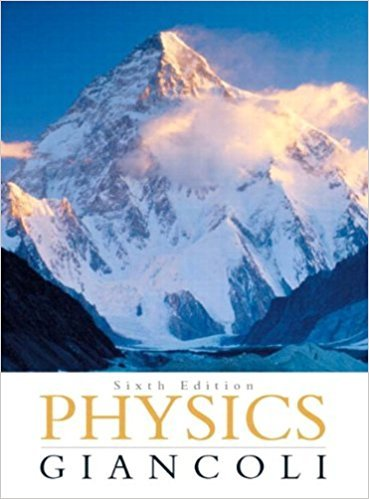×
×

# Solved: The gravitational force on the Moon due to theISBN: 9780321569837 99

## Solution for problem 16Q Chapter 5

Physics: Principles with Applications | 6th Edition

• Textbook Solutions
• 2901 Step-by-step solutions solved by professors and subject experts
• Get 24/7 help from StudySoup virtual teaching assistantsPhysics: Principles with Applications | 6th Edition

4 5 1 368 Reviews
12
0
Problem 16Q

The gravitational force on the Moon due to the Earth is only about half the force on the Moon due to the Sun. Why isn’t the Moon pulled away from the Earth?

Step-by-Step Solution:

Step 1 of 2</p>

We need to find out even the gravitational force on the Moon due to the Earth is only about half the force on the Moon due to the Sun, why isn’t the Moon pulled away from the Earth.

Step 2 of 2

##### ISBN: 9780321569837

The answer to “The gravitational force on the Moon due to the Earth is only about half the force on the Moon due to the Sun. Why isn’t the Moon pulled away from the Earth?” is broken down into a number of easy to follow steps, and 32 words. Since the solution to 16Q from 5 chapter was answered, more than 225 students have viewed the full step-by-step answer. This textbook survival guide was created for the textbook: Physics: Principles with Applications, edition: 6. This full solution covers the following key subjects: moon, EARTH, due, Force, half. This expansive textbook survival guide covers 33 chapters, and 4141 solutions. The full step-by-step solution to problem: 16Q from chapter: 5 was answered by , our top Physics solution expert on 09/09/17, 04:43AM. Physics: Principles with Applications was written by and is associated to the ISBN: 9780321569837.

Unlock Textbook Solution